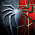11 Aug 2012

Tcl/Tk GUI Example with Variable Input by User

I recently used R with GUI-elements for the first time and browsed through the available online resources, but I didn't quite find what I was searching for: The user should be able to put in some variables and call a function with a button. In the end I did it with a little help from SO. Here is the working example that I eventually plugged together:

```require(tcltk)
mydialog <- function(){

xvar <- tclVar("")
yvar <- tclVar("")
zvar <- tclVar("")

tt <- tktoplevel()
tkwm.title(tt,"MYTEST")
x.entry <- tkentry(tt, textvariable=xvar)
y.entry <- tkentry(tt, textvariable=yvar)
z.entry <- tkentry(tt, textvariable=zvar)

reset <- function() {
tclvalue(xvar)<-""
tclvalue(yvar)<-""
tclvalue(zvar)<-""
}

reset.but <- tkbutton(tt, text="Reset", command=reset)

submit <- function() {
x <- as.numeric(tclvalue(xvar))
y <- as.numeric(tclvalue(yvar))
z <- as.numeric(tclvalue(zvar))
tkmessageBox(message=paste("x + y + z = ", x+y+z, ""))
}
submit.but <- tkbutton(tt, text="submit", command=submit)

quit.but <- tkbutton(tt, text = "Close Session",
command = function() {
q(save = "no")
tkdestroy(tt)
}
)

}

mydialog()
```

1.2.3.